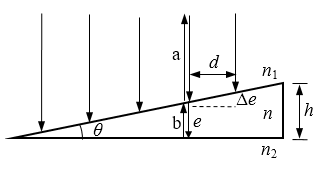# 劈尖的等厚干涉条纹

## 解析如图所示，劈尖的角度很小，真空波长为λ的单色光垂直入射到薄膜上时，产生反射光a和折射光b。b经过薄膜的下表面反射之后在上表面与a相遇。由于a、b两束光是同一束入射光分为两部分产生的，因而是相干光，相遇时可产生干涉条纹。一束光的强度分成两部分，这种产生干涉的方法称为分振幅法。

δ = 2ne + λ/2

δ = 2ne + λ/2 = kλ，(k = 1，2，3，…)

δ = 2ne + λ/2 = (2k + 1)λ/2，(k = 0，1，2，…)

k = 0时，e = 0，可知：劈尖的尖端是暗纹。同一条纹的劈尖厚度是相同的，因此这种干涉称为等厚干涉。干涉级次k越大，对应的厚度e也越大，相邻明纹或暗纹之间的厚度差为

Δe = λ/2n

$\theta \approx tan\theta = \frac{{\Delta e}}{d} = \frac{\lambda }{{2nd}}$

$h = kd\tan \theta = \frac{{k\lambda }}{{2n}}$

$I = {I_0}{\cos ^2}\frac{{\Delta \varphi }}{2} = {I_0}{\cos ^2}{\rm{(\pi }}\frac{\delta }{\lambda }) = {I_0}{\cos ^2}{\rm{[\pi (}}\frac{{2ne}}{\lambda } + \frac{1}{2})]$

## 图示

（1）如第1图所示，红光的干涉条纹是均匀分布的。干涉图样的最左边是尖劈的顶端，顶端出现暗条纹；图样共有10条明条纹。劈尖的角度为0.0072º，最大厚度为2.5微米。可见：利用劈尖干涉可以做精密测量。如第2图所示，绿光的干涉条纹也是均匀分布的，干涉条纹达到14条，这是因为绿光的波长比红光的波长短的缘故。如第3图所示，蓝光的干涉条纹仍然是均匀分布的，干涉条纹达到17条之多，这是因为蓝光的波长比绿光的波长还要短的缘故。

（2）如第4图所示，三种光混合后垂直照射劈尖，产生了彩色干涉条纹。最左边是三种光的暗纹，当劈尖的厚度增加时，三种光叠加在一起，形成白色条纹。由于红光的条纹最宽，所以在蓝光和绿光的暗条纹处出现红光的条纹。三种光的条纹错位叠加，就形成彩色条纹。

## 算法

%劈尖的等厚干涉条纹

%劈尖的等厚干涉条纹
clear                                  %清除变量
lambda=[750,540,440]*1e-9;             %波长
n=1.5;                                 %劈尖的折射率
k=10;                                  %明纹最高级次
dx=0.002;                              %明纹之间的距离
xm=k*dx;                               %劈尖的长度
x=linspace(0,xm,1000);                 %劈尖的长度向量
theta=lambda(1)/2/n/dx;                %劈尖的夹角
e=x*tan(theta);                        %劈尖的厚度向量
i1=cos(pi*(2*n*e/lambda(1)+1/2)).^2;   %红色反射光的相对光强（1）
M=zeros(1,length(x),3);                %1行若干列3页全零矩阵
M(:,:,1)=i1;                           %矩阵的红色页赋值（2）
figure                                 %开创图形窗口（3）
subplot(4,1,1)                         %选子图（3）
image(M)                               %画红色干涉条纹（3）
axis off                               %隐轴（3）
tit=['(\ith\rm=',num2str(e(end)*1000),'mm'];%厚度文本
tit=[tit,',\it\theta\rm=',num2str(theta*180/pi),'\circ)'];%角度文本
fs=16;                                 %字体大小
title(['红光在劈尖上的等厚干涉条纹',tit],'FontSize',fs)%标题

i2=cos(pi*(2*n*e/lambda(2)+1/2)).^2;   %绿色反射光的相对光强（4）
M=zeros(1,length(x),3);                %1行若干列3页全零矩阵（4）
M(:,:,2)=i2;                           %矩阵的绿色页赋值（4）
subplot(4,1,2)                         %选子图（4）
image(M)                               %画绿色干涉条纹（4）
axis off                               %隐轴（4）
title('绿光在劈尖上的等厚干涉条纹','FontSize',fs)%标题
i3=cos(pi*(2*n*e/lambda(3)+1/2)).^2;   %蓝色反射光的相对光强（5）
M=zeros(1,length(x),3);                %1行若干列3页全零矩阵（5）
M(:,:,3)=i3;                           %矩阵的蓝色页赋值（5）
subplot(4,1,3)                         %选子图（5）
image(M)                               %画蓝色干涉条纹（5）
axis off                               %隐轴（5）
title('蓝光在劈尖上的等厚干涉条纹','FontSize',fs)%标题
M(:,:,2)=i2;                           %矩阵的绿色页赋值（6）
M(:,:,1)=i1;                           %矩阵的红色页赋值（6）
subplot(4,1,4)                         %选子图（6）
image(M)                               %画彩色干涉条纹（6）
axis off                               %隐轴（6）
title('白光在劈尖上的等厚干涉条纹','FontSize',fs)%标题

## 说明

（1）先计算红光的光强。

（2）矩阵的前二维表示坐标，第三维的第1页表示红色。

（3）图像指令image可根据三维矩阵画干涉条纹。由于只有第1页有光强的数据，所以画红色的干涉图样。注意：image指令中的参数是矩阵。

（4）矩阵的第2页表示绿色，当第2页有不为零的数据时，image指令就只画绿色的干涉条纹。

（5）第3页表示蓝色，当第3页有不为零的数据时，image指令就只画蓝色的干涉条纹。

（6）当3页都有不为零的数据时，image指令就画各种颜色的干涉条纹。这种将单色光强通过矩阵合成复色光强的方法可称为光强的矩阵合成法。

2006 - 2023，推荐分辨率1024*768以上，推荐浏览器Chrome、Edge等现代浏览器，截止2021年12月5日的访问次数：1872万9823 站长邮箱